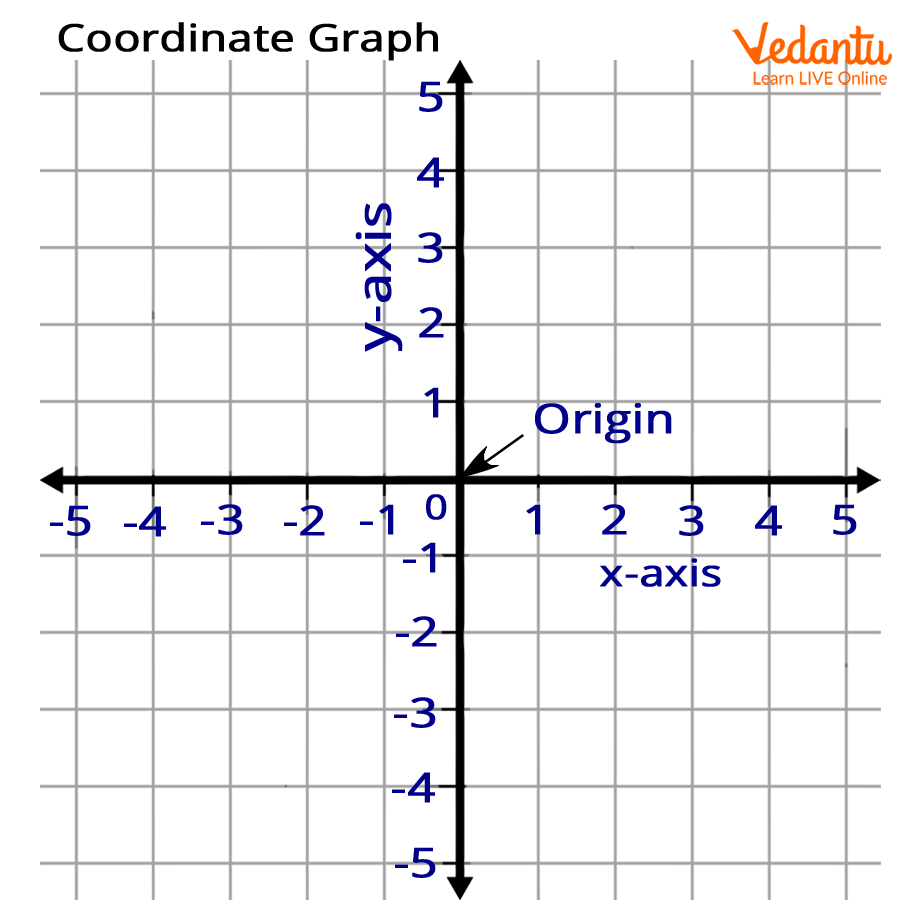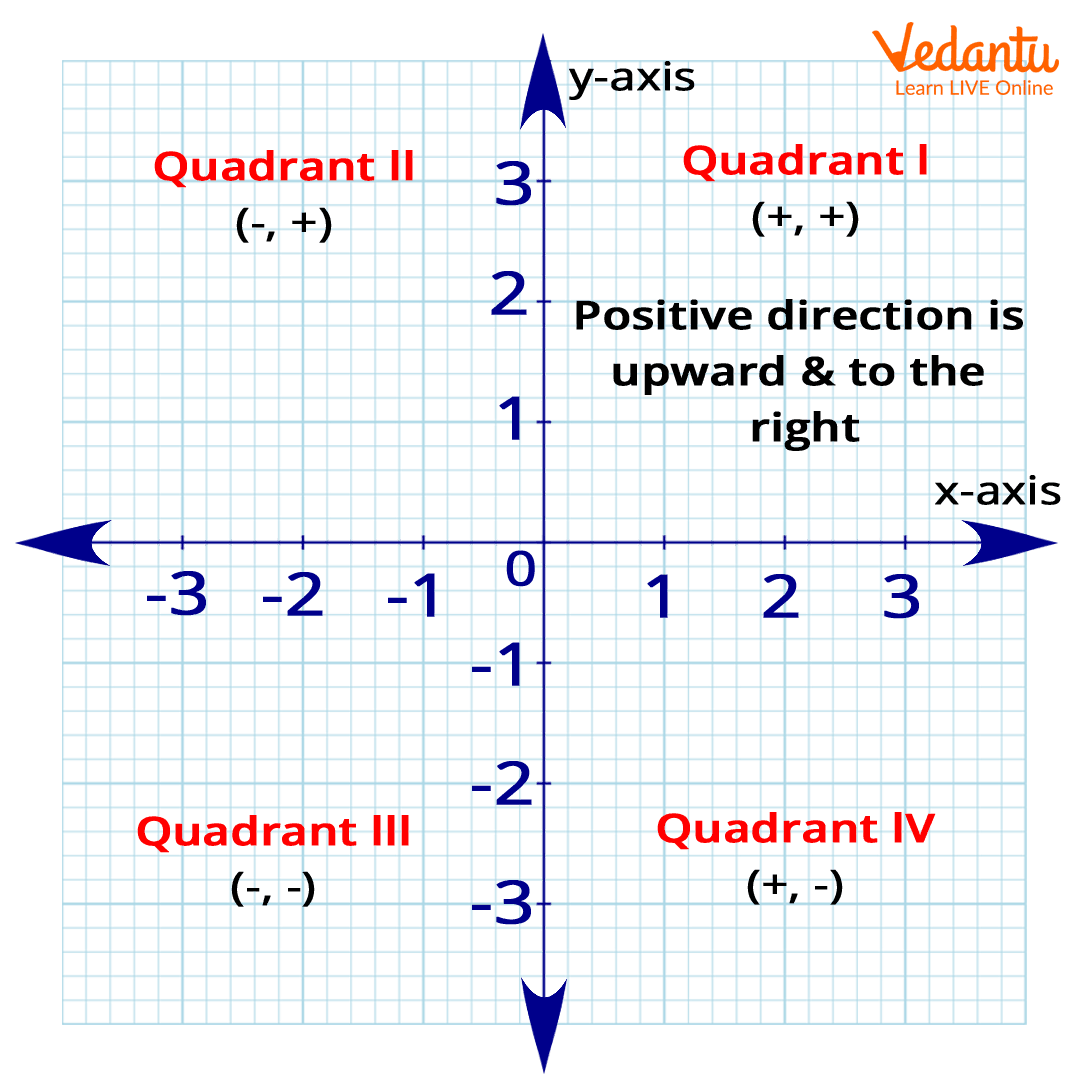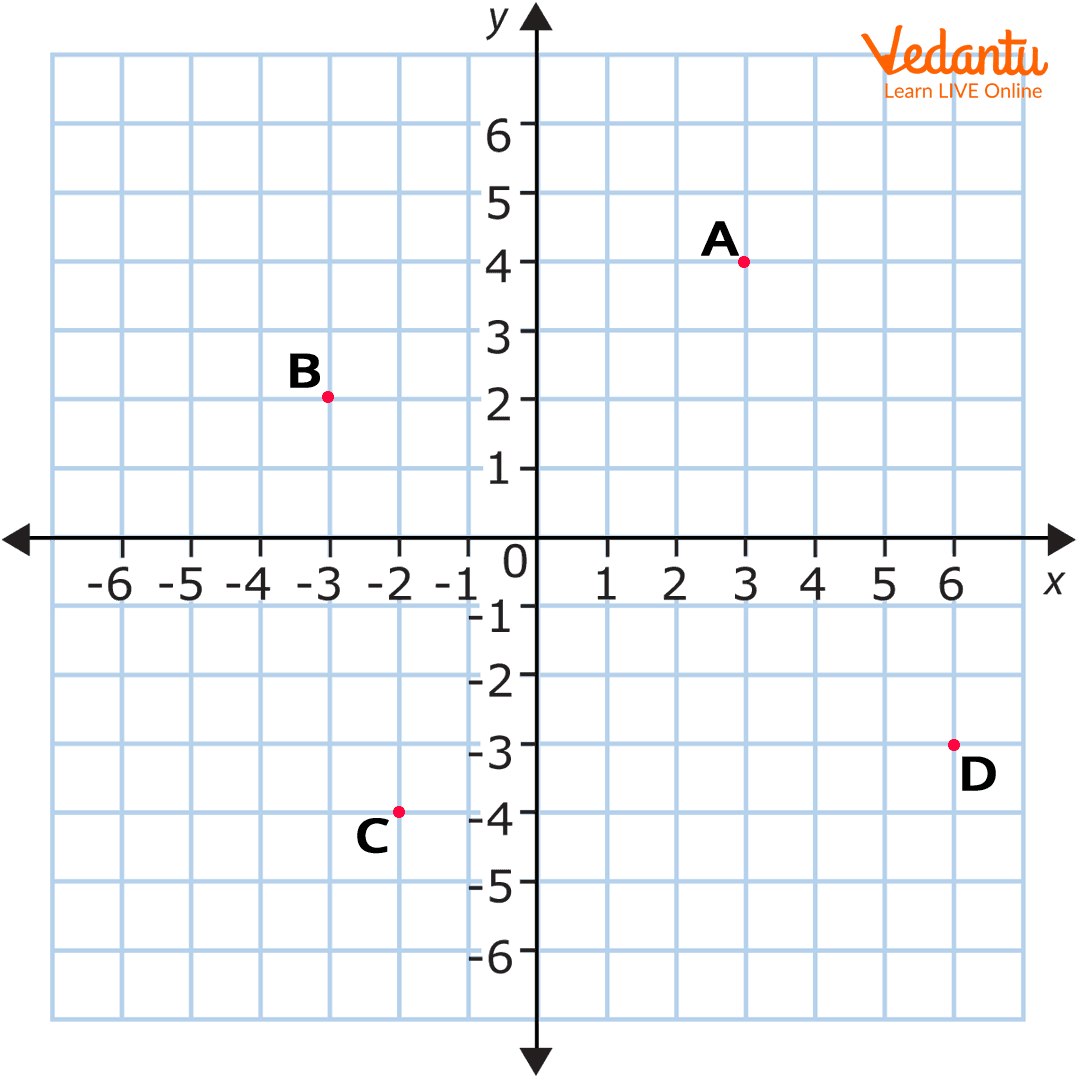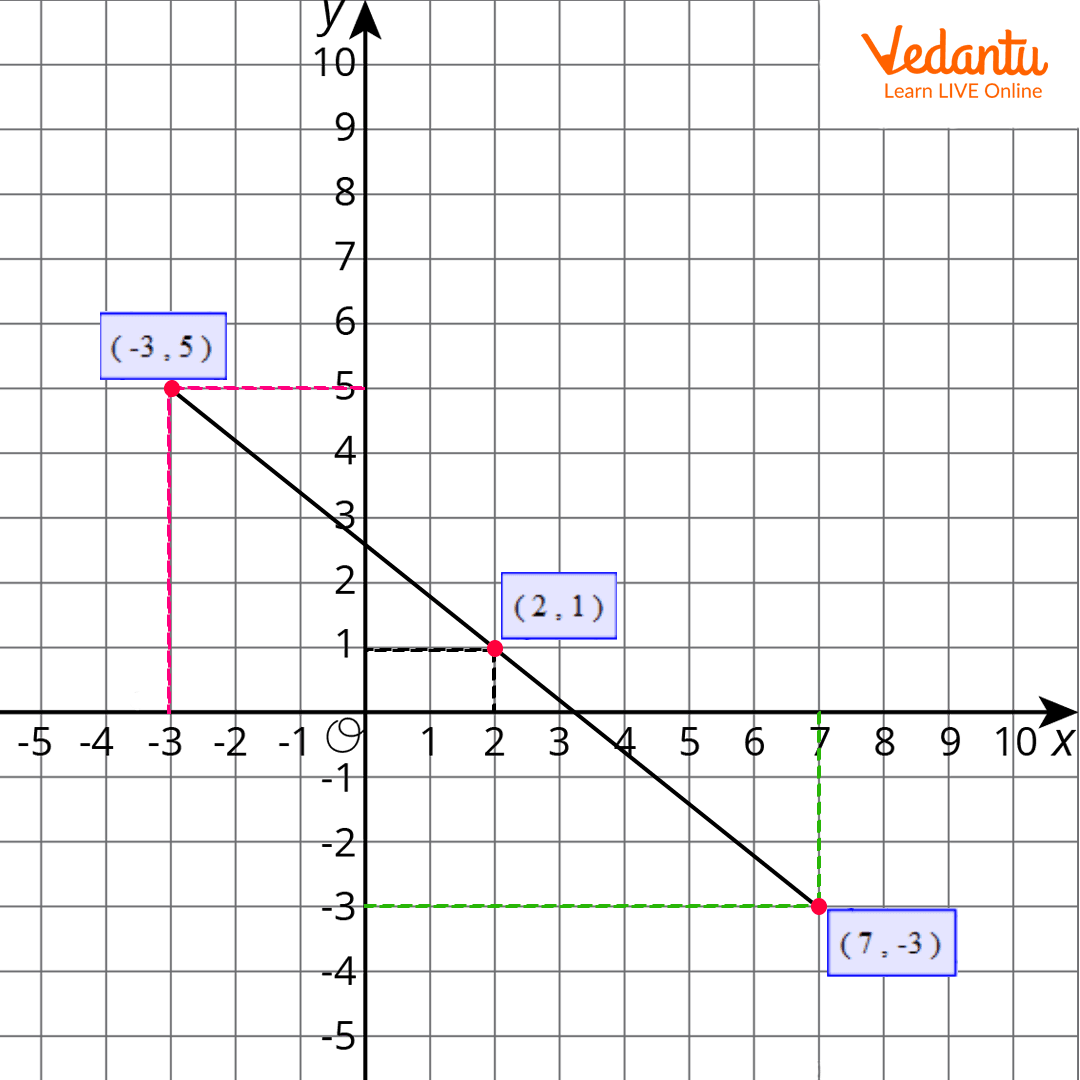Courses
Courses for Kids
Free study material
Offline Centres
More

# Coordinate GridLast updated date: 29th Nov 2023
Total views: 158.7k
Views today: 2.58k## What is a Coordinate Plane?

A coordinate plane is a two-dimensional surface made up of two number lines. It is formed when a horizontal line known as the X-axis and a vertical line known as the Y-axis intersect at the origin. Points are located using the numbers on a coordinate grid. A coordinate plane is useful for graphing points, lines, and other objects. It functions as a map, providing precise directions from one point to another.## Description of a Coordinate Plane

In this section, we will learn about the components of a coordinate plane.

Axis - There are two axes in a coordinate plane. The horizontal line is called the x-axis and the vertical line is called the y-axis.

Quadrant I - This quadrant comes in the positive - positive part, i.e., the x-axis is positive and the y-axis is also positive.

Quadrant II - This quadrant comes in the negative - positive part, i.e., the x-axis is negative and the y-axis is positive.

Quadrant III - The third quadrant comes in the negative - negative part, i.e., both the x-axis and y-axis are negative.

Quadrant IV - The fourth quadrant comes in the positive - negative quadrant, i.e., the x-axis is positive and the y-axis is negative.

Positive x-axis - The horizontal line towards the right from the origin is the positive x-axis.

Negative x-axis - The horizontal line towards the left from the origin is the negative x-axis.

Positive x-axis - The vertical line above the origin is the positive y-axis.

Negative x-axis - The vertical line below the origin is the negative y-axis.

Coordinates - The points that are located on the x and y axes or the coordinate graph are called the coordinates. It is always written in a particular format, which is (x,y).

Here is an image to understand the coordinate graph in a better way:## How to Locate a Point on the Coordinate Plane?

Here is the step by step explanation of locating a point on the coordinate plane:

2. Find the quadrant by checking the sign of the coordinates. For example - (2,3) will be on the quadrant I, (-4,5) will be on quadrant II, (-3,-2) will be on the quadrant III, and (6,-3) will be on the quadrant IV.

3. Once you have found the coordinate, locate the point on the x-axis on the right quadrant. For example - Point A on the first quadrant has coordinate 3 on the x-axis.1. Similarly, locate the point on the y-axis on the right quadrant. For example - On the above image, point A on the first quadrant has coordinate 4 on the y-axis.

2. The point is located. For the above image, point A has the coordinate of (3,4).

## How to Draw the Coordinate Graph?

Here is the step by step explanation of how to draw the graph:

Let us consider the following points: (-3,5), (2,1), and (7,-3)

1. Identify the quadrants of the coordinates. For the first coordinates (-3,5), the quadrant will be quadrant II, for the second coordinates (2,1), the quadrant will be quadrant I, and for the third coordinates (7,-3), the quadrant will be quadrant IV.

2. Find the points on the right quadrant on the x-axis and y-axis.

3. Join the points to form a straight line.

4. Consider the following image for your reference:## Quick Fact

 The coordinate plane was introduced by French mathematician René Descartes. It is after his name that coordinate plane is also called Cartesian coordinate system.

## Conclusion

So far you have learned about the coordinate graph, about its components, how to locate a point on the coordinate graph, and how to draw the graph. If you want to know more details about the coordinate graphs and solve exercises, you can explore more on our website. Apart from the coordinate graphs, we have covered all other mathematical topics. So you can explore them anytime on our website and enjoy learning!

## FAQs on Coordinate Grid

1. What is a coordinate grid? What is it called?

A coordinate grid is a graphical representation of intersection of two lines - one horizontal and one vertical, called the x-axis and y-axis. It is called cartesian plane or coordinate plane.

2. Where do we draw the coordinate grid?

We draw a coordinate grid on graph paper. It is all about the measurement, so drawing a coordinate grid on a graph paper is a must.